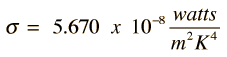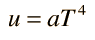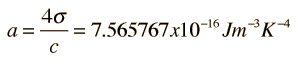When the electromagnetic radiation in a region of space is at equilibrium with its surroundings, it can be described by the Planck radiation formula. The total energy radiated from an area in this region of space is given by the Stefan-Boltzmann law and the energy density associated with the radiation can be related to that law. In the development of the expression for the radiation from a hot surface, it was found that the radiated power is related to the energy density by the factor c/4 .Where does the factor c/4 come from?

The Stefan-Boltzmann law can then be related to the energy density by this factor, and the cumulative energy density for all wavelengths of radiation can be expressed aswhereFor a region of space where the temperature is T = K,

the radiation energy density is x 10^ J/m3.

In astronomy and astrophysics texts, the energy density in radiation is typically writtenwhere a is called the radiation constant and has the valueDivision of energy between radiation and matter
Index